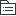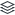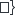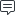# 复盘

152
|
23

## 相关模板推荐

•## 复盘 —— 作品大纲

•复盘思路
• GRAI
• Goal（目标回顾）
• Result（结果陈述）
• Analysis（过程分析）
• Insight（归类总结）
• 四部框架
• 回顾目标
• 当初的目的或期望是什么
• 评估结果
• 和原定目标相比有哪些亮点和不足
• 分析原因
• 事情成功和失败的根本原因
• 主观
• 客观
• 总结规律
• 更有效/符合本质的规律
• 实施哪些新举措
• 继续哪些措施等
• 复盘误区
• 自己骗自己
• 不狠心剖析自己
• 流于形式
• 走过场
• 为总结而总结
• 追究责任
• 人身攻击
• 批斗大会
• 强调客观
• 推卸责任
• 忽视主观的意义
• 急于得出结论
• 不考虑全盘
• 草草结案
•九部复盘法
• 选择复盘内容
• 近期工作重点
• 已完结
• 项目的某个部分
• 可以是全案
• 未完结
• 项目某个模块
• 自己参与
• 自己全负责
• 部分流程
• 和他人多沟通
• 团队内部
• 其他负责人
• 相关的同事
• 外部视角
• 上游合作者
• 下游承接者
•争取做到覆盖全面
• 覆盖面小
• 自己工作某细节
• 整理材料
• 操作过程全部材料
• 设计稿、文案、使用截图、用户反馈等
• 反复迭代的记录
•要全面、有代表性
• 数据
• 过程中的数据
• 最终的数据
•反映真实情况
• 文档
• 排期
• 功能点的优先级
• 开发时长
• 测试文件等
• 列出当初的目标
• 最初制定的目标
• 归纳完善的目标
• 整理不足的目标
•完整的列出来
• 列出目标完成情况
• 目标是希望达成的，结果是实际做到的
• 列出目标后进行结果比对
• 预定目标达到
• 预定目标超越
• 预定目标失败
• 过程中新增了其他目标
• 过程中放弃了原有的目标
• 叙述执行的过程
• 想象场景
• 做到白居易的老妪能懂
• 不评判、不分析
• 只阐述
• 尽可能的详细、客观
• 且能用别人听得懂
• 听得下去的语言
• 工作日志非常重要
• 当天发生什么
• 当天数据如何
• 当时想法怎样
• 看记录知状态
• 发现细节调整状态
• 自我剖析
• 自我剖析实质
• 用今天的眼光和能力去审视昨天的做法
• 从昨天的行为中学到对未来有用的信息
• 要注意
• 客观公正
• 不给自己留情面
• 参考角度
• 连续追问找根源
• 失败
• 中间出了什么问题？
• 当时如何应对的？
• 应对效果如何？
• 成功
• 其中的关键点是什么？
• 有哪些优势条件？
• 是否存在偶然性？
• 发现差异取原因
• 对比往期结果
• 横向
• 纵向
• 同行业其他公司
• 分析原因明内外
• 自己努力带来的？
• 外在环境造成的？
• 厘清线索定可控
• 可控？
• 是否做到了最好？
• 不可控？
• 是否提前沟通/了解？
• 是否过程敦促？
• 半可控？
• 自己能掌握的部分做好了吗？
• 给别人留出发挥的空间了吗？
• 给别人提供及时的帮助了吗？
•两个基本认知
• 哪些没尽力？
• 哪些出过力？
• 哪些无着力？
•不可控
• 自我成长的关键
• 自己去分析事情的过程
• 审视自身
• 当时思考和处理
• 开放心胸
• 听取意见
• 实现“求内
• 未来能力的组成
• 得出观点
• 关于本质
• 关于规律
• 工作本质
• 重点
• 难点
• 核心
• 本质
• 经得起设问
• 不同人提问项目各方面
• 突破项目本身
• 突破认识局限
• 达到深层思考
• 当初目标怎么制定？
• 为何决定做
• 用什么方式做
• 制定怎样的目标
• 复盘>总结
• 通过推演
• 找不同可能性
• 找成功和失败的边界
• 审视“如何确事定”
• 获得自己、团队成长
•成长的关键
• 执行的怎么样？
• 最受关注是细节
• 该挖掘细节背后的细节
• 如何对执行进行规定？
• 执行过程是否严格按计划？
• 过程出现意外不能避免吗？
• 哪些是需要注意的新情况？
• 为何一定要这样？
• 凡是都有最基本的前提和假设
• 做知识付费
• 够量的付费用户
• 符合用户需求的课
• 用户能通过路径购买、消费
• 通过追问前提和假设
• 找到“论据”
• 追溯动机
• 思考“还有其他可能性吗？”
• 总结规律
• 前七步为了得出
• 符合逻辑关系的
• 一般通用性
• 具体解释性
• 实际指导性
•客观规律
• 整理归档
• 案例佐证
• 适用于当下场合
• 其他事能够验证
• 其他行业现象
• 保存起来
• 资料文件
• 思考过程
• 经验
• 规律
•便于以后更好使用